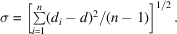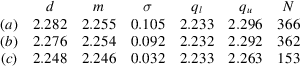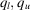International
Tables for
Crystallography
Volume C
Mathematical, physical and chemical tables
Edited by E. Prince

International Tables for Crystallography (2006). Vol. C, ch. 9.6, pp. 813-814

## Section 9.6.2.4. Statistics

A. G. Orpen,a L. Brammer,b F. H. Allen,c D. G. Watsonc and R. Taylorc

aSchool of Chemistry, University of Bristol, Bristol BS8 1TS, England,bDepartment of Chemistry, University of Missouri–St Louis, 8001 Natural Bridge Road, St Louis, MO 63121-4499, USA, and cCambridge Crystallographic Data Centre, 12 Union Road, Cambridge CB2 1EZ, England

#### 9.6.2.4. Statistics

| top | pdf |

Where there are less than four independent observations of a given bond length, then each individual observation is given explicitly in Table 9.6.3.3. In all other cases, the following statistics were generated by the program STATS.

 (i) The unweighted sample mean, d, whereandis the ith observation of the bond length in a total sample of n observations. Recent work (Taylor & Kennard, 1983, 1985, 1986) has shown that the unweighted mean is an acceptable (even preferable) alternative to the weighted mean, where the ith observation is assigned a weight equal to 1/var(di). This is especially true where structures have been pre-screened on the basis of precision. (ii) The sample median, m. This has the property that half of the observations in the sample exceed m, and half fall short of it. (iii) The sample standard deviation, σ, where(iv) The lower quartile for the sample,. This has the property that 25% of the observations are less thanand 75% exceed it. (v) The upper quartile for the sample,. This has the property that 25% of the observations exceedand 75% fall short of it. (vi) The number (n) of observations in the sample.

The statistics given in Table 9.6.3.3correspond to distributions for which the automatic 4σ cut-off (see above) had been applied, and any manual removal of additional outliers (an infrequent operation) had been performed. In practice, a very small percentage of observations were excluded by these methods. The major effect of removing outliers is to improve the sample standard deviation, as shown in Fig. 9.6.2.1(b)in which four (out of 366) observations are deleted.Figure 9.6.2.1 | top | pdf |Effects of outlier removal and subdivision based on coordination number and oxidation state. Cu—Cl: (a) all data; (b) all data without outliers [> 4σ (sample) from mean]; (c) all data for which Cu is 4-coordinate, CuII.The statistics chosen for tabulation effectively describe the distribution of bond lengths in each case. For a symmetrical, normal distribution, the mean (d) will be approximately equal to the median (m), the lower and upper quartiles () will be approximately symmetric about the median, and 95% of the observations may be expected to lie within ±2σ of the mean value. For a skewed distribution, d and m may differ appreciably andandwill be asymmetric with respect to m. When a bond-length distribution is negatively skewed, i.e. very short values are more common than very long values, then it may be due to thermal-motion effects; the distances used to prepare the table were not corrected for thermal libration.

In a number of cases, the initial bond-length distribution was clearly not unimodal as in Fig. 9.6.2.1(a). Where possible, such distributions were resolved into their unimodal components (as in Fig. 9.6.2.1c) on chemical or structural criteria. The case illustrated in Fig. 9.6.2.1, for Cu—Cl bonds, is one of the most spectacular examples, owing to the dramatic consequences of oxidation state and coordination number (and Jahn–Teller effects) on the structures of copper complexes.

### References

Taylor, R. & Kennard, O. (1983). The estimation of average molecular dimensions from crystallographic data. Acta Cryst. B39, 517–525.Google Scholar
Taylor, R. & Kennard, O. (1985). The estimation of average molecular dimensions. 2. Hypothesis testing with weighted and unweighted means. Acta Cryst. A41, 85–89.Google Scholar
Taylor, R. & Kennard, O. (1986). Cambridge Crystallographic Data Centre. 7. Estimating average molecular dimensions from the Cambridge Structural Database. J. Chem. Inf. Comput. Sci. 26, 28–32.Google Scholar